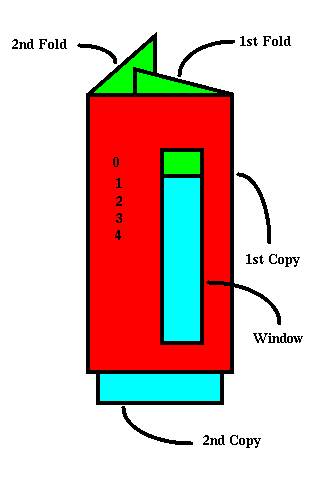# Pinhole Exposure Determination

I determine the exposure to use for my pinhole photos by using a Pentax digital spot meter (although any method of determining the base exposure is OK) and a pinhole calculator. The calculator is used to multiply the exposure time from the meter (which is normally set to f/8) to allow for the focal ratio of the pinhole camera (f/228). After determining the pinhole exposure I usually add one stop (double the exposure time) for reciprocity failure in the film I use.

There are several calculators you can use to adjust the exposure time. Andreas Irmer has kindly allowed me to distribute his disk shaped Exposure Value Extension calculator. You will need a postscript printer (or postscript translator like Ghostscript) to print it out. The instructions for using the calculator are on the printed page.

After seeing Andreas Irmer's disk calculator I decided to try making my own. This also needs a postscript printer, and I was lazy so you will need to print two copies in order to cut out both disks. This calculator works like Andreas Irmer's, but it isn't as nice looking.

If you have a regular electronic calculator (or are just good at multiplying in your head) you could multiply the exposure time from the meter by 1024. This assumes that the meter was set at f/8 and the pinhole is f/256 (1/3 f-stop off from f/228). Different apertures (either for the meter setting or the pinhole) would require different multipliers. For example, for a meter setting of f/16 the multiplier would be 256.

My original pinhole caculator was made after seeing (and making) a paper slide rule. The pinhole exposure calculator is assembled from two copies of a table of f-stops and exposure times. The first column is just the line number. The second column is focal ratio and the third column is exposure time. The focal ratios and exposure times change by 1/3 f-stop from one line to the next.

To make the calculator print two copies of the table. Take the first copy and cut out the column that contains the exposure times creating a window that is the width and height of that column. Then fold the paper vertically just to the right of the window. Make a second vertical fold just to the left of the first column forming a flat tube. You'll have to trim the paper so that the flaps will lie on top of each other. Tape the two flaps together. Next take the second copy of the table and cut out the three columns so that they will fit inside the first (folded) copy, and the exposure column is visible. The figure below should make things clear.To use the calculator slide the inner paper until your exposure meter reading lines up (e.g., 1/4 second, f/8) and then look down the focal ratio column until you find your aperture and read off the exposure time in the window (e.g., 3:23, f/228).